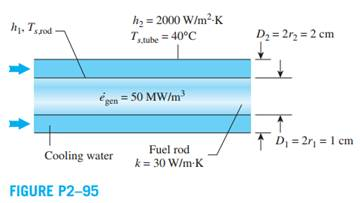Create an Account

Home / Questions / A cylindrical nuclear fuel rod of 1 cm in diameter is encased in a concentric tube of 2 cm...

A cylindrical nuclear fuel rod of 1 cm in diameter is encased in a concentric tube of 2 cm in diameter where cooling water flows through the annular region between the fuel rod

A cylindrical nuclear fuel rod of 1 cm in diameter is encased in a concentric tube of 2 cm in diameter, where cooling water flows through the annular region between the fuel rod (k = 30 W/m·K) and the concentric tube. Heat is generated uniformly in the rod at a rate of 50 MW/m3. The convection heat transfer coefficient for the concentric tube surface is 2000 W/m2 ·K. If the surface temperature of the concentric tube is 40°C, determine the average temperature of the cooling water. Can one use the given information to determine the surface temperature of the fuel rod? Explain.Jul 21 2020 View more View LessSubscribe To Get Solution